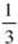﻿ ﻿Answers and Explanations - Trigonometric Functions and Their Inverses - Functions - REVIEW OF MAJOR TOPICS - SAT SUBJECT TEST MATH LEVEL 2

## PART 2## REVIEW OF MAJOR TOPICS## CHAPTER 1Functions

###1.3 Trigonometric Functions and Their InversesDefinitions

1. (A) Reference angle is 40°. Cosine in quadrant IV is positive.

2. (D) See corresponding figure. Therefore, sin.3. (D) Angleis in quadrant II since sec < 0 and sin > 0. Therefore, tan.4. (A) Cofunctions of complementary angles are equal. x – 30 + x = 90 finds a reference angle of 60° for x. The angle in quadrant III that has a reference angle of 60° is 240°.

5. (A) Angleis in quadrant II, and sinis positive. Angleis in quadrant IV, and sinis negative.

* 6. (E) Put your calculator in degree mode, cos 310° + cos 190°0.643 + (–0.985)– 0.342. Checking the answer choices shows that –cos 70°–0.342.

Arcs and Angles

1. (E).

2. (D)* 3. (C)* 4. (A)≈ 0.7

s = r≈ 1(0.7) ≈ 0.7.

* 5. (C) Change 25° to 0.436 radian.

s = r, and so 12 = r(0.436) and r = 27.5 inches.

Special Angles

1. (A) Sketch a –60° angle in standard position as shown in the figure below.The tangent ratio is2. (C) Sketch an angle ofradians in standard position, as shown in the figure below.The cosine ratio is.

3. (E) First, determine an angle between 0° and 360° that is coterminal with 540° by subtracting 360° from 540° repeatedly until the result is in this interval. In this case, one subtraction suffices. Since coterminal angles have the same trig values, csc 540° = csc 180°. Sketch the figure belowIn a quadrantal angle r = 1, and the cosecant ratio is, which is undefined.

Graphs

1. (C) Period. Point P isof the way through the period. Amplitude is 1 because the coefficient of sin is 1. Therefore, point P is at.

2. (E) Amplitude =. Period =. Graph translatedunit up. Graph looks like a cosine graph reflected about x-axis and shifted upunit.

* 3. (C) Graph 4cosusing ZOOM/ZTRIG and observe that the portion of the graph betweenis decreasing.* 4. (C) Graph the function and determine its maximum (2) and minimum (–2). Subtract and then divide by 2.

5. (D) Period =.

* 6. (C) Graph the function using 0 for Xmin andfor Xmax. Observe that the maximum occurs when x =. Then.

7. (D) Period(from the figure), so M =. Phase shift for a sine curve in the figure is –. Therefore,x + N = 0 when x = –. Therefore,Identities, Equations, and Inequalities

* 1. (A) sin 2x = 2 sin x cos.

2. (D) Since tangent and cotangent are cofunctions, tan A = cot(90° – A), so B = 90° – A, and A + B = 90°.

* 3. (D)4. (A) sin 74° = 2 sin 37° cos 37°. Since. Since 74° is in the first quadrant, the positive square root applies, so cos.

* 5. (B).

* 6. (A) Graph y = sin x and y = cos in radian mode using the Xmin = 0 and Xmax =. Observe that the first graph is beneath the second on [0,0.79].

7. (D) Remember that the range of the sine function is [–1,1], so the second term ranges from 6 to –6.

Inverse Trig Functions

* 1. (E) Set your calculator to degree mode, and enter 2nd sin–1.

* 2. (D) Set your calculator to radian mode, and enter 2nd cos–1(–0.5624).

* 3. (B) Set your calculator to degree mode, and enter 2nd tan–1(tan 128°).

* 4. (E) The range of inverse cotangent functions consists of only positive numbers.

5. (A) Since, I is true. Since cos–11 = 0 and cos–1(–1) =, II is not true. Since the range of cos–1 is [0,], III is not true because cos–1 can never be negative.

6. (E) 3x = arccos, and so x =arccos.

Triangles

1. (D) Law of Sines:. The figure shows two possible locations for B, labeled B1 and B2, where mAB1C = 45° and mAB2C = 135°. Corresponding to these, mACB1 = 105° and mACB2 = 15°. Of these, only 15° is an answer choice.* 2. (E) By the Law of Sines:. The figure below shows this to be an ambiguous case (an angle, the side opposite, and another side), soor C = 180° – 41.81° = 138.19°.3. (A) The angles are 15°, 45°, and 120°. Let c be the longest side and b the next longest.* 4. (D) Use the Law of Cosines. Let the sides be 4, 5, and 6. 16 = 25 + 36 – 60 cos A. Cos, which implies that* 5. (C) Law of Cosines: d2 = 36 + 64 – 96 cos 120°. d2 = 148. Therefore, d12.6. (D) Altitude to base= 8 sin 60° = 4. Therefore, 4< a < 8.

* 7. (D) A = 45°. Law of Sines:. Therefore, a = 1014.

8. (D) Area. Therefore, C = 60° or 120°.

9. (C) Area. C = 60° or 120°. Use Law of Cosines with 60° and then with 120°.

Note: At this point in the solution you know there have to be two values for C. Therefore, the answer must be Choice C or E. If C = 10 (from Choice E), ABC is a right triangle with area =· 6 · 8 = 24. Therefore, Choice E is not the answer, and so Choice C is the correct answer.

10. (A) In I the altitudeand so 2 triangles.so only 1 triangle. In III the altitudeso no triangle.

﻿